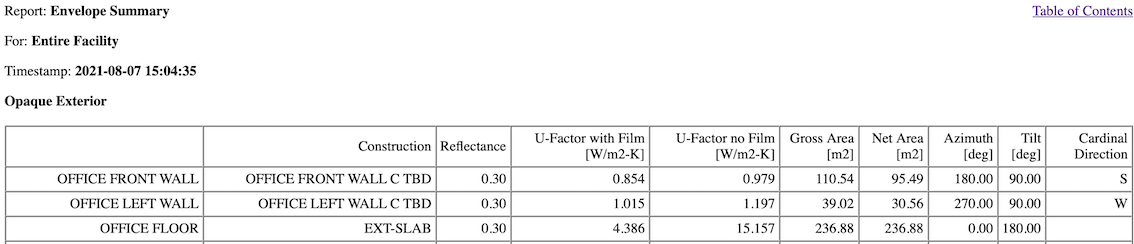Question-and-Answer Resource for the Building Energy Modeling Community
Get s tarted with the Help page

# Finding R-values of constructions in OpenStudio

Hello. I would like to find the R-values of constructions such as wall, floor, roof, and attic. Each of these constructions is composed of several layers of materials. Is there a way to output their R-values? Perhaps there is an existing OS measure? Or is there a way to calculate based on the material thermal properties? Thank you!

edit retag close merge delete

Sort by » oldest newest most voted

As an alternative to @Denis Bourgeois's answer if you want to get this without running the simulation OpenStudio has a method layered_construction. thermalConductance that will return this thermal conductance. It is used in some of our measures to report on the initial and then modified R values.

Here is a use of it in the extension gem that ships with OpenStudio. This implementation adds film coefficient

std = Standard.build(target_standard)
film_coefficients_r_value = std.film_coefficients_r_value(intended_surface_type, includes_int_film = true, includes_ext_film = true)
thermal_conductance = surface_detail[:construction].thermalConductance.get
r_value_with_film = 1 / thermal_conductance + film_coefficients_r_value
source_units = 'm^2*K/W'
target_units = 'ft^2*h*R/Btu'
r_value_ip = OpenStudio.convert(r_value_with_film, source_units, target_units).get

more

If you successfully ran an EnergyPlus simulation, you can retrieve construction U-factors from the /reports/eplustbl.html Envelope Summary Tables (here, an excerpt from the US DOE Commercial Prototype Warehouse):You can alternatively just add up the layer R-values (material thickness / material conductivity).

more